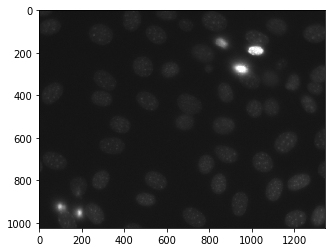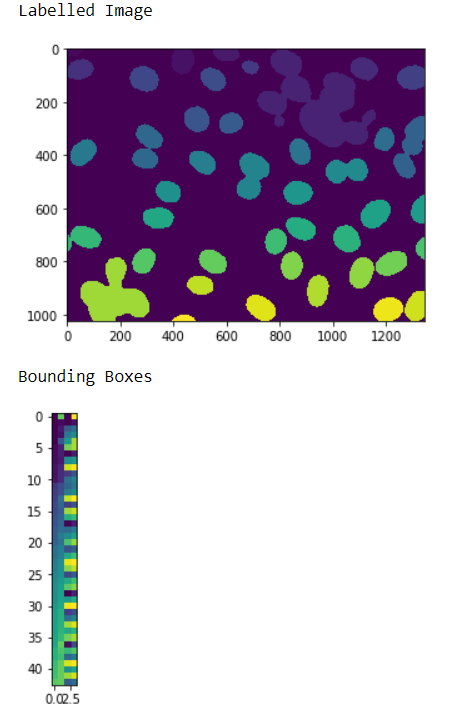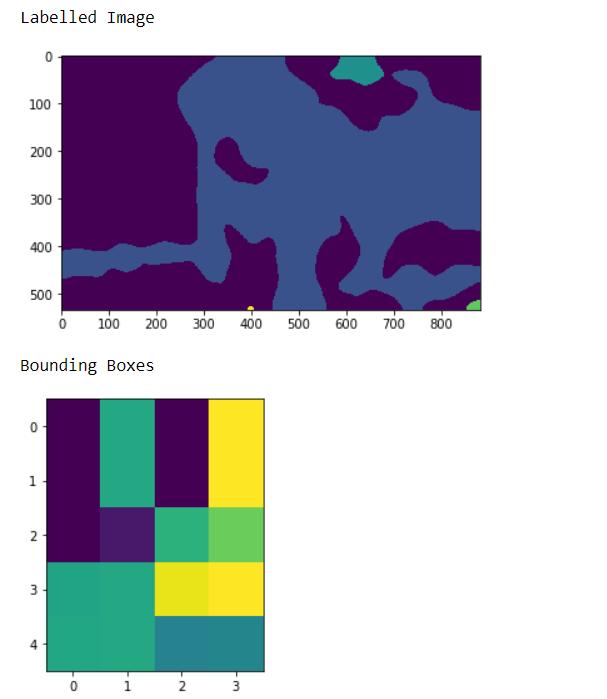# Mahotas – Getting Bounding Boxes of Labelled Image

• Last Updated : 16 May, 2022

In this article we will see how we can get the bounding boxes of all the objects in the labelled image in mahotas. For this we are going to use the fluorescent microscopy image from a nuclear segmentation benchmark. We can get the image with the help of command given below

`mhotas.demos.nuclear_image()`

Below is the nuclear_imageIn order to do this we will use mahotas.labelled.bbox method

Syntax : mahotas.labelled.bbox(labelled_image)
Argument : It takes numpy.ndarray object as argument i.e labelled image
Return : It returns numpy.ndarray object i.e bounding box image

Note : The input of the this should  be the filtered image object which is labeled
In order to filter the image we will take the image object which is numpy.ndarray and filter it with the help of indexing, below is the command to do this

`image = image[:, :, 0]`

Example 1 :

## Python3

 `# importing required libraries``import` `mahotas``import` `numpy as np``from` `pylab ``import` `imshow, show``import` `os` `# loading nuclear image``f ``=` `mahotas.demos.load(``'nuclear'``)` `# setting filter to the image``f ``=` `f[:, :, ``0``]` `# setting gaussian filter``f ``=` `mahotas.gaussian_filter(f, ``4``)` `# setting threshold value``f ``=` `(f> f.mean())` `# creating a labelled image``labelled, n_nucleus ``=` `mahotas.label(f)`  `# showing the labelled image``print``(``"Labelled Image"``)``imshow(labelled)``show()` `# getting bounding boxes``relabeled ``=` `mahotas.labelled.bbox(labelled)` `# showing the image``print``(``"Bounding Boxes"``)``imshow(relabelled)``show()`

Output :Example 2 :

## Python3

 `# importing required libraries``import` `numpy as np``import` `mahotas``from` `pylab ``import` `imshow, show`` ` `# loading image``img ``=` `mahotas.imread(``'dog_image.png'``)``   ` `# filtering the image``img ``=` `img[:, :, ``0``]``    ` `# setting gaussian filter``gaussian ``=` `mahotas.gaussian_filter(img, ``15``)`` ` `# setting threshold value``gaussian ``=` `(gaussian > gaussian.mean())`` ` `# creating a labelled image``labelled, n_nucleus ``=` `mahotas.label(gaussian)``  ` `print``(``"Labelled Image"``)``# showing the gaussian filter``imshow(labelled)``show()`` ` `# getting bounding boxes``relabelled ``=` `mahotas.labelled.bbox(labelled)` `# showing the image``print``(``"Bounding Boxes"``)``imshow(relabelled)``show()`

Output :My Personal Notes arrow_drop_up